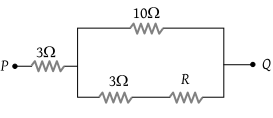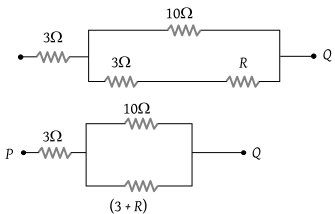# NEET Questions Solved

In the circuit shown here, what is the value of the unknown resistor R so that the total resistance of the circuit between points P and Q is also equal to(1) 3 ohms

(2) $\sqrt{39}\text{\hspace{0.17em}}ohms$

(3) $\sqrt{69}\text{\hspace{0.17em}}ohms$

(4) 10 ohms

(3) The given circuit can be simplified as follows$R=3+\frac{10×\left(3+R\right)}{10+3+R}=3+\frac{30+10R}{13+R}$

$R=\frac{39+3R+30+10R}{13+R}=\frac{69+13R}{13+R}$

$13R+{R}^{2}=69+13R$

$R=\sqrt{69}\Omega$.

Difficulty Level:

• 9%
• 26%
• 56%
• 10%